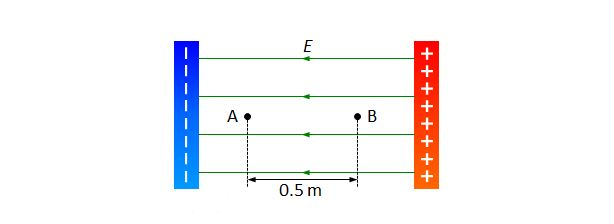# Electric potential between 2 charged platesA uniform electric field is formed between two charged plates as shown above. The electric field strength is $0.2 \text{ N/C}$. If the distance between the two points A and B is $0.5 \text{ m}$, then what is the electric potential difference between A and B?

×